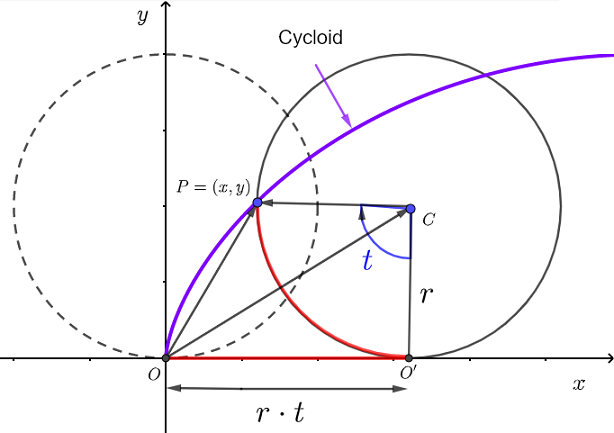# Parametric Equations of Cycloid

## Parametric Equations

A wheel touches a flat surface at point $$P$$ assumed to be a fixed point on the wheel. As the wheel, with radius $$r$$, rotates around its center on the flat surface (without sliding) , point $$P$$ describes a curve called a cycloid . (see video below)

What the video shows is explained in Fig. 1 below: point $$P$$ a fixed point on the wheel rotate around the center of the rotating wheel and move horizontally and vertically taking position $$P_1$$, $$P_2$$ ...

In Fig.2 below, is shown point $$P$$ afer a rotation of the wheel around its center $$C$$ measured by angle $$t$$ made by $$CP$$ and $$CA$$ such that $$CA$$ is parallel to the $$y$$ axis. Hence the components of
vector $$CP$$ are given by
$\vec {CP} = \; \left\lt r \cos \theta \; , \; r \sin \theta \; \right\gt \qquad (I)$ where $$\theta$$ is the angle in standard position.
We need to express the components of $$\vec {CP}$$ in terms of angle $$t$$ noting that
$$\theta = \dfrac{3\pi}{2} - t$$
Substitute $$\theta$$ in (I) and write
$\vec {CP} = \; \left\lt r \; \cos \left(\dfrac{3\pi}{2} - t\right) \; , \; r \; \sin \left(\dfrac{3\pi}{2} - t\right) \; \right\gt$
Use the
trigonometric formulas $$\quad \cos(A - B) = \cos A \cos B + \sin A \sin B$$ and $$\quad \sin(A - B) = \sin A \cos B - \cos A \sin B$$ to expand the above and simplify using $$\cos \left(\dfrac{3\pi}{2}\right) = 0$$ and $$\sin \left(\dfrac{3\pi}{2} \right) = -1$$ to obtain
$\vec {CP} = \left\lt \; - r \; \sin t \; , \; - r \; \cos t \; \right\gt$Fig.2 - Coordinates of a Point on a Wheel with Respect to the Center of the Wheel

In order to find the equation of the cycloid, we need to find the coordinates of point $$P$$ with respect to the origin $$O$$ of the coordinate system.Fig.3 - Coordinates of a Point on a Wheel with Respect to the Origin of the Origin of the System of Axes
After a rotation of angle $$t$$, the $$x$$ coordinate $$O O'$$ of the center $$C$$ of the circle is equal to the length of the arc $$O'P$$ which is givn by $r \; t$ $$t$$ in radians
The $$y$$ coordinate $$O'C$$ of the center $$C$$ is equal to $$r$$
Hence the components of vector $$OC$$ is given by
$\vec {OC} = \left\lt \; r \; t \; , \; r \; \right\gt$
Vector $$OP$$ is given as a sum of vectors as follows $\vec {OP} = \vec {OC} + \vec {CP}$ We substitute the components of vectors $$\vec {OC}$$ and $$\vec {CP}$$ found above to obtain $\vec{OP} = \left\lt \; r \; t \; - r \; \sin t \; , \; r \; - r \; \cos t \right\gt$ The coordinates of $$P$$ are given by the
parametric equations \begin{equation} \left\{ \begin{aligned} x(t) & = r(t - \sin t) \\\\ y(t) & = r(1 - \cos t) \end{aligned} \right. \end{equation} where $$t$$ is the angle, in radians, through which the wheel has rotated around its center. Angle $$t$$ is measured starting from the negative y axis in clockwise direction.

## Length of a Cycle of a Cycloid

The length $$L$$ of a curve whose equation is $$y = f(x)$$ is given by $L = \int_{x_1}^{x_2} \sqrt {1+\left(\dfrac{dy}{dx} \right)^2} dx$ The derivative $$\dfrac{dy}{dx}$$ of parametric equations is given by $\dfrac{dy}{dx} = \dfrac{\dfrac{dy}{dt}}{\dfrac{dx}{dt}}$ and $$dx = \dfrac{dx}{dt} dt$$ in the above to obtain $L = \int_{t_1}^{t_2} \sqrt {1+\left( \dfrac{\dfrac{dy}{dt}}{\dfrac{dx}{dt}} \right)^2} \dfrac{dx}{dt} dt \qquad (II)$ which may be written as $L = \int_{t_1}^{t_2} \dfrac{1}{\left|\dfrac{dx}{dt}\right|} \sqrt { \left( \dfrac{dx}{dt}\right)^2 +\left( \dfrac{dy}{dt} \right)^2} \dfrac{dx}{dt} dt$ One cycle of a cycloid corresponds to one rotation of the wheel and hence the limits integraion may be selected as $$t_1 = 0$$ and $$t_2 = 2 \pi$$
using the parametric equations of the cycloid, the drivatives with respect to $$t$$ are given by $\dfrac{dx}{dt} = r(1-\cos t )$ and $\dfrac{dy}{dt} = r \sin t$ and note that $$\dfrac{dx}{dt} = r(1-\cos t )$$ is non negative quantity and hence the simplification $\left|\dfrac{dx}{dt}\right| = \dfrac{dx}{dt}$ Substitute the above in $$II$$ to simplify the formula for $$L$$ as follows $L = \int_{0}^{2 \pi} \sqrt { \left( \dfrac{dx}{dt}\right)^2 +\left( \dfrac{dy}{dt} \right)^2} dt$ Substitute $$\dfrac{dx}{dt}$$ and $$\dfrac{dy}{dt}$$ by their expressions above and simplify to obtain $L = \int_{0}^{2 \pi} \sqrt { \left( r(1-\cos t ) \right)^2 +\left( r \sin t \right)^2} dt$ $$r$$ is a constant and hence $$L$$ may be written as $L = r \int_{0}^{2 \pi} \sqrt { \left( 1 + \cos^2 t - 2 \cos t + \sin^2 t \right)} dt$ Use the trigonometric identity $$\sin^2 t + \cos^2 t = 1$$ to simplify $L = r \int_{0}^{2 \pi} \sqrt { 2 \left ( 1 - \cos t \right)} dt$ Use the trigonometric identity $$\quad 1 - \cos t = 2 \sin^2 (t/2)$$ and write $L = r \int_{0}^{2 \pi} \sqrt { 4 \left ( \sin^2 (t/2) \right)} dt$ which may be written as $L = 2 r \int_{0}^{2 \pi} \left| \sin (t/2) \right| dt$ $$\sin (t/2)$$ is non negative on the interval $$[0 , 2 \pi ]$$ and we therefore write $L = 2 r \int_{0}^{2 \pi} \sin (t/2) dt$ Evaluate the above integral $L = 2 r \left[-2\cos \left(\frac{t}{2}\right)\right]_0^{2\pi}$ $L = 4 r [- \cos \pi + \cos 0 ]$ The length of one cycle of a cycloid is given by $\boxed {L = 8 r }$